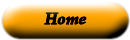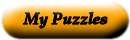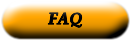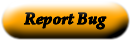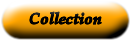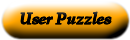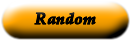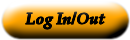slope matching sides of 2 or more polygons corresponding angles figures with the same shape but not necessarily the same size are similar scale ratios that name the same comparison quadrant a rate in which the 2nd quantity in the comparison is 1 unit y-axis the technique of using similar figures and proportions to find a measure similar angles in the same position formed when a third line intersects 2 lines cross product the product of numbers on the diagonal when comparing 2 ratios scale drawing the point where the x-axis intersect on the coordinate plane (0,0) coordinate plane an equation that states that 2 ratios are equivalent scale factor a proportional model is a 3-dimensional object Indirect measurement a drawing that uses a scale to make an object smaller than or larger than the real object origin the vertical axis on a coordinate plane corresponding sides a ratio that compares 2 quantities measured in different units unit rate the horizontal axis on a coordinate plane rate a plane formed but he intersection of a horizontal number line called the x-axis and a vertical number line called the y-axis scale model the ratio between 2 sets of measurements x-axis a pair of numbers that can be used to locate the point on a coordinate plane proportion a measure of the steepness of a line on a graph; the rise divided by the run ordered pair the x- and y-axises divide the coordinate plane into 4 regions. equivalent ratios the ratio used to enlarge or reduce similar figures

Use the "Printable HTML" button to get a clean page, in either HTML or PDF, that you can use your browser's print button to print. This page won't have buttons or ads, just your puzzle. The PDF format allows the web site to know how large a printer page is, and the fonts are scaled to fill the page. The PDF takes awhile to generate. Don't panic!Web armoredpenguin.com